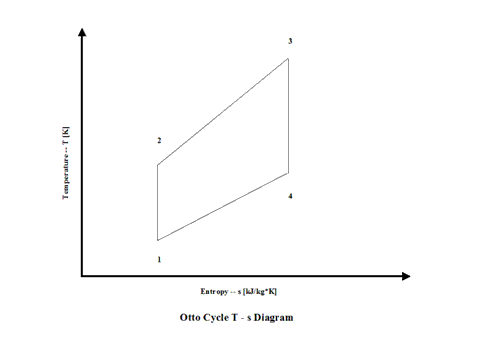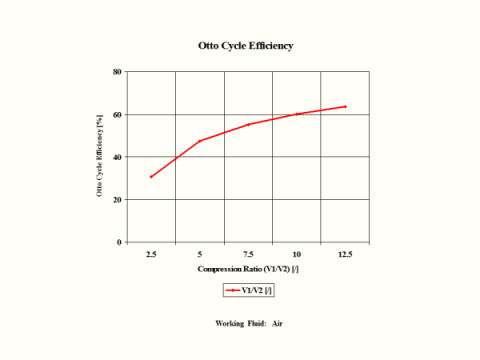### Otto Cycle Analysis -- Free One (1) Credit Hour of CE

Otto Cycle

Introduction

This section provides an Otto Cycle analysis when the working fluid is air.

Analysis

In the presented Otto Cycle analysis, only air is considered as the working fluid behaving as a perfect gas -- specific heat has a constant value.  Ideal gas state equation is valid -- pv = RT.

Air enters a cylinder at point 1 when compression starts and it ends at point 2.  Isentropic compression is considered with no entropy change.  Heat addition starts at point 2 and it ends at point 3.  At a constant volume, air gets heated and the working fluid temperature raises.  Expansion starts at point 3 and it ends at point 4.  Isentropic expansion is considered with no entropy change.  Air heat rejection starts at point 4 and it ends at point 1.   At a constant volume, air gets cooled and the working fluid temperature decreases.  It should be mentioned that air at point 1 enters the compression process again and the cycle is repeated.

Figure 1 contains an Otto Cycle pressure vs volume diagram.Figure 1 - Otto Cycle Pressure vs Volume Diagram

Figure 2 presents an Otto Cycle temperature vs entropy diagram.Figure 2 - Otto Cycle Temperature vs Entropy Diagram

Figure 3 presents the Otto Cycle efficiency as a function of the compression ratio.  It should be noted that the inlet conditions are standard ambient conditions:  temperature of 298 [K] and absolute pressure of 1 [atm].Figure 3 - Otto Cycle Efficiency

Figure 4 presents the Otto Cycle power output as a function of the combustion temperature and compression ratio.  It should be noted that the number of revolutions is 60 [1/s] for given geometry of the four cylinder and four stroke Otto engine.Figure 4 - Otto Cycle Power  Output

One can notice that the Otto Cycle efficiency increases with an increase in the compression ratio values.  One can notice that the Otto Cycle power output increases with an increase in the combustion temperature.  The Otto Cycle power output is greater for the higher compression ratio values.

Assumptions

Working fluid is air.  There is no friction.  Compression and expansion are isentropic -- there is no entropy change.  Ideal gas state equation is valid -- pv = RT.  Air behaves as a perfect gas -- specific heat has a constant value.

Governing Equations

T2/T1 = (V1/V2)^(ϰ-1)
V
1/V2 = (T2/T1)^1/(
ϰ-1)

T3/T4 = (V4/V3)^(ϰ-1)
V
4/V3 = (T3/T4)^1/(
ϰ-1)
ϰ = cp/cv
cp - cv = R
pv = RT
w = q
h - ql
qh = cv(T3 - T2)
q
l = cv(T4 - T1)
w = c
v(T3 - T2) - cv(T4 - T1)
W = (c
v(T3 - T2) - cv(T4 - T1))m
efficiency = 1 - 1/compression ratio^(
ϰ-1)
compression ratio = V
1/V2

Input Data

T1 = 298 [K]
p
1 = 1 [atm]
T
3 =  1,200, 1,500 and 1,800 [K]

compression ratio = 2.5, 5, 7.5, 10 and 12.5 [/]
R = 0.2867 [kJ/kg*K]
c
p = 1.004 [kJ/kg*K]

ϰ = 1.4 [/]

Results

Otto Cycle Efficiency vs Compression Ratio

Compression
Ratio
[/]

2.5

5

7.5

10

12.5

Otto Cycle
Efficiency
[%]

30.7

47.5

55.3

60.2

63.6

Otto Cycle Power Output

Power Output
[kW]

Compression Ratio
[/]

5

15

Combustion
Temperature
[K]

1,200

167.1

151.2

1,500

246.4

251.7

1,800

325.6

352.2

Conclusions

The Otto Cycle efficiency increases with an increase in the compression ratio values.  Also, the Otto Cycle power output increases with an increase in the combustion temperature.  The Otto Cycle power output is greater for the higher compression ratio values.

References

JANAF Thermochemical Data - Tables, 1970